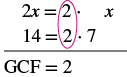## Finding the Greatest Common Factor of a Polynomial

### Learning Outcomes

• Factor the greatest common monomial out of a polynomial

Factor a PolynomialOne of these things is not like the others.

Before we solve polynomial equations, we will practice finding the greatest common factor of a polynomial. If you can find common factors for each term of a polynomial, then you can factor it, and solving will be easier.

To help you practice finding common factors, identify factors that the terms of the polynomial have in common in the table below.

Polynomial Terms Common Factors
$6x+9$ $6x$ and $9$ $3$ is a factor of $6x$ and  $9$
$a^{2}–2a$ $a^{2}$ and $−2a$ a is a factor of $a^{2}$ and $−2a$
$4c^{3}+4c$ $4c^{3}$ and $4c$ $4$ and c are factors of $4c^{3}$ and  $4c$

Remember that you can multiply a polynomial by a monomial as follows:

$\begin{array}{ccc}\hfill 2\left(x + 7\right)&\text{factors}\hfill \\ \hfill 2\cdot x + 2\cdot 7\hfill \\ \hfill 2x + 14&\text{product}\hfill \end{array}$

Here, we will start with a product, like $2x+14$, and end with its factors, $2\left(x+7\right)$. To do this we apply the Distributive Property “in reverse”.

To factor a polynomial, first identify the greatest common factor of the terms. You can then use the distributive property to rewrite the polynomial in a factored form. Recall that the distributive property of multiplication over addition states that a product of a number and a sum is the same as the sum of the products.

### Distributive Property

If $a,b,c$ are real numbers, then

$a\left(b+c\right)=ab+ac\text{ and }ab+ac=a\left(b+c\right)$

#### Distributive Property Forward and Backward

Forward: Product of a number and a sum: $a\left(b+c\right)=a\cdot{b}+a\cdot{c}$. You can say that “$a$ is being distributed over $b+c$.”

Backward: Sum of the products: $a\cdot{b}+a\cdot{c}=a\left(b+c\right)$. Here you can say that “a is being factored out.”

We first learned that we could distribute a factor over a sum or difference, now we are learning that we can “undo” the distributive property with factoring.

So how do we use the Distributive Property to factor a polynomial? We find the GCF of all the terms and write the polynomial as a product!

### example

Factor: $2x+14$

Solution

 Step 1: Find the GCF of all the terms of the polynomial. Find the GCF of $2x$ and $14$.Step 2: Rewrite each term as a product using the GCF. Rewrite $2x$ and $14$ as products of their GCF, $2$. $2x=2\cdot x$ $14=2\cdot 7$ $2x+14$ $\color{red}{2}\cdot x+\color{red}{2}\cdot7$ Step 3: Use the Distributive Property ‘in reverse’ to factor the expression. $2\left(x+7\right)$ Step 4: Check by multiplying the factors. Check: $2(x+7)$ $2\cdot{x}+2\cdot{7}$ $2x+14\quad\checkmark$

### try it

Notice that in the example, we used the word factor as both a noun and a verb:

$\begin{array}{cccc}\text{Noun}\hfill & & & 7\text{ is a factor of }14\hfill \\ \text{Verb}\hfill & & & \text{factor }2\text{ from }2x+14\hfill \end{array}$

### Factor the greatest common factor from a polynomial

1. Find the GCF of all the terms of the polynomial.
2. Rewrite each term as a product using the GCF.
3. Use the Distributive Property ‘in reverse’ to factor the expression.
4. Check by multiplying the factors.

Notice in the next example how, when we factor 3 out of the expression, we are left with a factor of 1.

### example

Factor: $3a+3$

### try it

The expressions in the next example have several prime factors in common. Remember to write the GCF as the product of all the common factors.

### example

Factor: $12x - 60$

### try it

Watch the following video to see more examples of factoring the GCF from a binomial.

Now we’ll factor the greatest common factor from a trinomial. We start by finding the GCF of all three terms.

### example

Factor: $3{y}^{2}+6y+9$

### try it

In the next example, we factor a variable from a binomial.

### example

Factor: $6{x}^{2}+5x$

### try it

When there are several common factors, as we’ll see in the next two examples, good organization and neat work helps!

### example

Factor: $4{x}^{3}-20{x}^{2}$

### Example

Factor $25b^{3}+10b^{2}$.

The factored form of the polynomial $25b^{3}+10b^{2}$ is $5b^{2}\left(5b+2\right)$. You can check this by doing the multiplication. $5b^{2}\left(5b+2\right)=25b^{3}+10b^{2}$.

Note that if you do not factor the greatest common factor at first, you can continue factoring, rather than start all over.

For example:

$\begin{array}{l}25b^{3}+10b^{2}=5\left(5b^{3}+2b^{2}\right)\,\,\,\,\,\,\,\,\,\,\,\text{Factor out }5.\\\,\,\,\,\,\,\,\,\,\,\,\,\,\,\,\,\,\,\,\,\,\,\,\,\,\,\,\,\,=5b^{2}\left(5b+2\right) \,\,\,\,\,\,\,\,\,\,\,\,\,\,\,\text{Factor out }b^{2}.\end{array}$

Notice that you arrive at the same simplified form whether you factor out the GCF immediately or if you pull out factors individually.

### example

Factor: $21{y}^{2}+35y$

### example

Factor: $14{x}^{3}+8{x}^{2}-10x$

### try it

When the leading coefficient, the coefficient of the first term, is negative, we factor the negative out as part of the GCF.

### example

Factor: $-9y - 27$

### try it

Pay close attention to the signs of the terms in the next example.

### example

Factor: $-4{a}^{2}+16a$

### TRY IT

This next example shows factoring a binomial when there are two different variables in the expression.

### Example

Factor $81c^{3}d+45c^{2}d^{2}$.

The following video provides two more examples of finding the greatest common factor of a binomial

This last example shows finding the greatest common factors of trinomials.

## Summary

A whole number, monomial, or polynomial can be expressed as a product of factors. You can use some of the same logic that you apply to factoring integers to factoring polynomials. To factor a polynomial, first identify the greatest common factor of the terms, and then apply the distributive property to rewrite the expression. Once a polynomial in $a\cdot{b}+a\cdot{c}$ form has been rewritten as $a\left(b+c\right)$, where a is the GCF, the polynomial is in factored form.

## Contribute!

Did you have an idea for improving this content? We’d love your input.